Question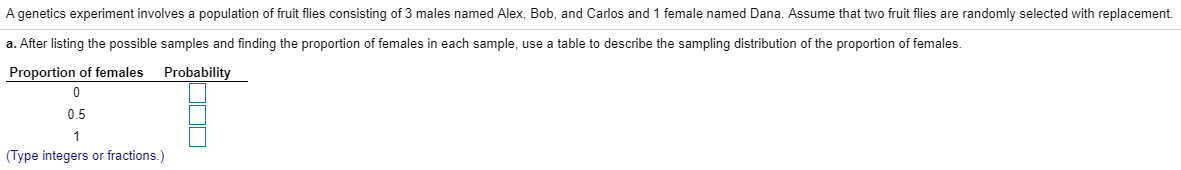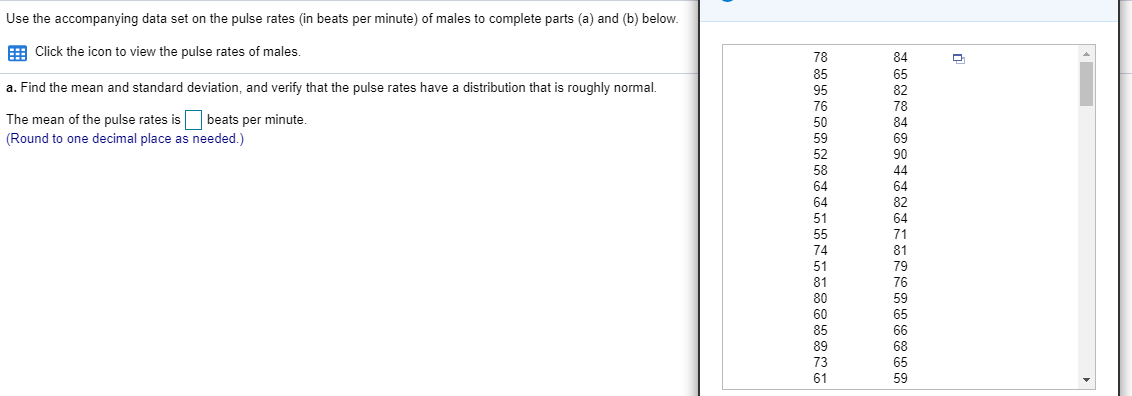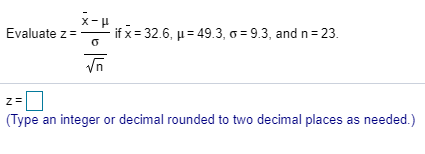We would be looking at the first question all parts here as:

Q1) We are given here that there are 3 males and 1 female.

As we are drawing with replacement here, therefore the probability of getting a female remains same in both the draws given as:
p = P(female) = n(Females) / n(Total) = 1/(1 + 3) = 1/ 4 = 0.25

Therefore the number of females in the 2 draws here could be modelled as: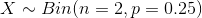The probability distribution table now is computed here as:

 Proportion of Females Probability 0 P(X = 0) = (1 - 0.25)2 = 0.5625 0.5 P(X = 1) = 2*0.25*(1 - 0.25) = 0.375 1 P(X = 2) = 0.252 = 0.0625

These are the required probabilities here.

#### Earn Coins

Coins can be redeemed for fabulous gifts.

Similar Homework Help Questions
• ### Use the accompanying data set on the pulse rates (in beats per minute) of males to...Use the accompanying data set on the pulse rates (in beats per minute) of males to complete parts (a) and (b) below. Click the icon to view the pulse rates of males. a. Find the mean and standard deviation, and verify that the pulse rates have a distribution that is roughly normal. The mean of the pulse rates is beats per minute. (Round to one decimal place as needed.) 78 85 95 76 50 59 52 58 64 64 51...

• ### Use the accompanying data set on the pulse rates​ (in beats per​ minute) of males to...

Use the accompanying data set on the pulse rates​ (in beats per​ minute) of males to complete parts​ (a) and​ (b) below. LOADING... Click the icon to view the pulse rates of males. a. Find the mean and standard​ deviation, and verify that the pulse rates have a distribution that is roughly normal. The mean of the pulse rates is 71.871.8 beats per minute. ​(Round to one decimal place as​ needed.) The standard deviation of the pulse rates is 12.212.2...

• ### A genetics experiment involves a population of fruit flies consisting of 1 male named Andre and 3 females named Barbara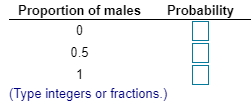A genetics experiment involves a population of fruit flies consisting of 1 male named Andre and 3 females named Barbara, Carla, and Diana. Assume that two fruit flies are randomly selected with replacement. a. After listing the possible samples and finding the proportion of males in each sample, use a table to describe the sampling distribution of the proportion of males.

• ### x Refer to the accompanying data set and construct a 90% confidence interval estimate of the...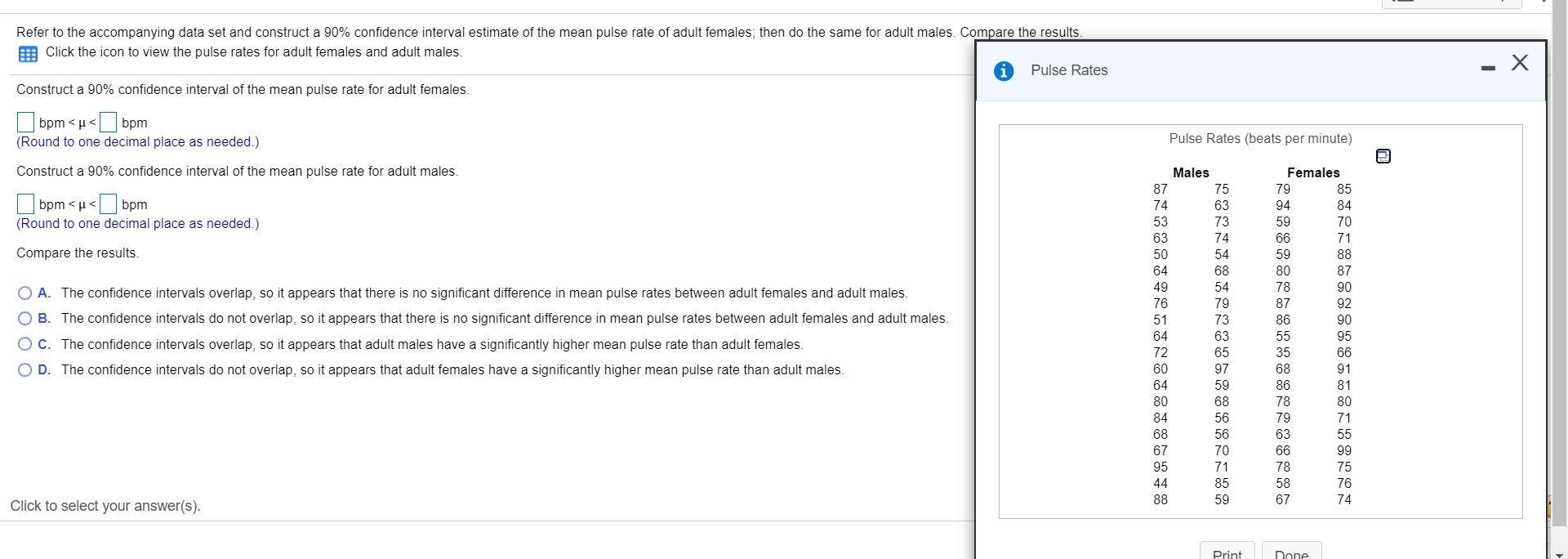x Refer to the accompanying data set and construct a 90% confidence interval estimate of the mean pulse rate of adult females, then do the same for adult males. Compare the results. Click the icon to view the pulse rates for adult females and adult males. Pulse Rates Construct a 90% confidence interval of the mean pulse rate for adult females. | bpm<<bpm (Round to one decimal place as needed.) Pulse Rates (beats per minute) e Construct a 90% confidence...

• ### Refer to the accompanying data set and construct a 90% confidence interval estimate of the mean...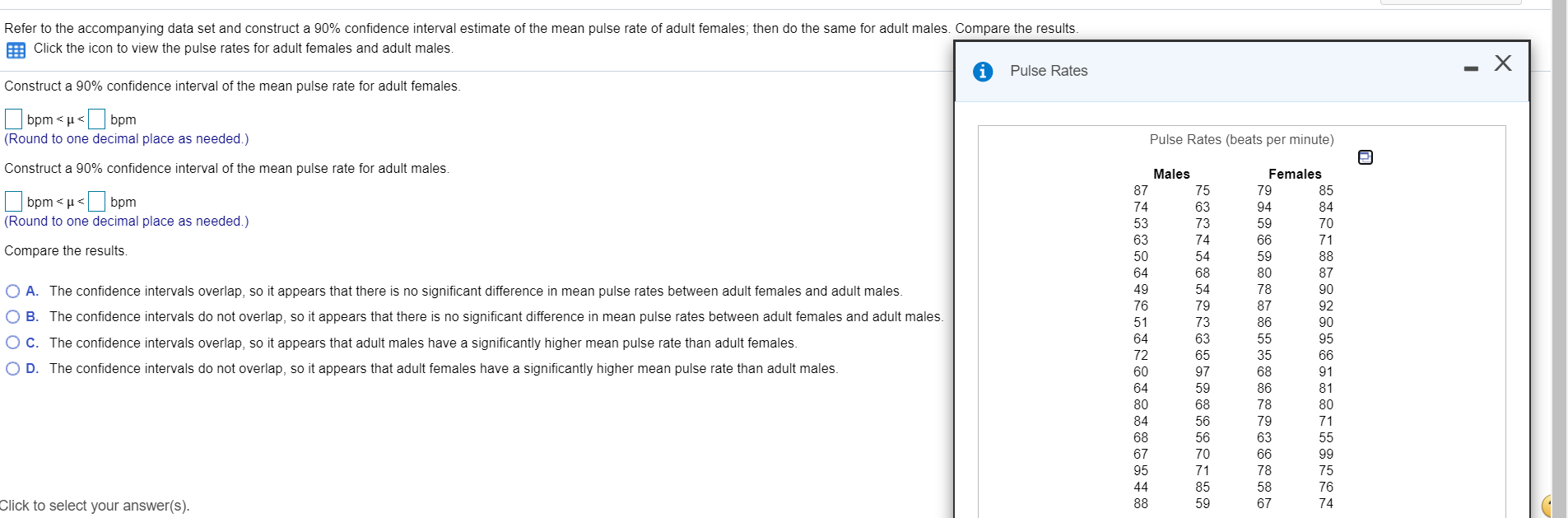Refer to the accompanying data set and construct a 90% confidence interval estimate of the mean pulse rate of adult females; then do the same for adult males. Compare the results. Click the icon to view the pulse rates for adult females and adult males. Pulse Rates Construct a 90% confidence interval of the mean pulse rate for adult females. x bpm<u<bpm (Round to one decimal place as needed.) Pulse Rates (beats per minute) Construct a 90% confidence interval of...

• ### please explain answer Listed below are pulse rates (beats per minute) from samples of adult males...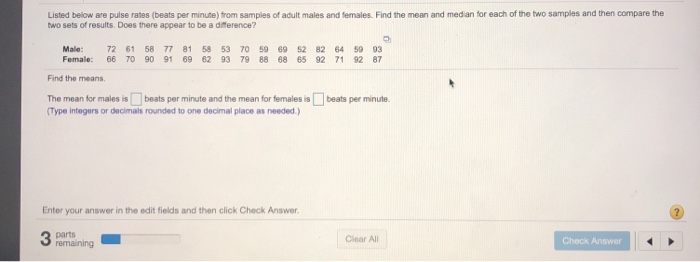please explain answer Listed below are pulse rates (beats per minute) from samples of adult males and females. Find the mean and median for each of the two samples and then compare the two sets of results. Does there appear to be a difference? Male: 72 61 58 77 81 58 53 70 59 69 52 82 64 59 93 Female: 66 70 90 91 69 62 93 79 88 68 65 92 71 92 87 Find the means The...

• ### Listed below are pulse rates (beats per minute) from samples of adult males and females. Find...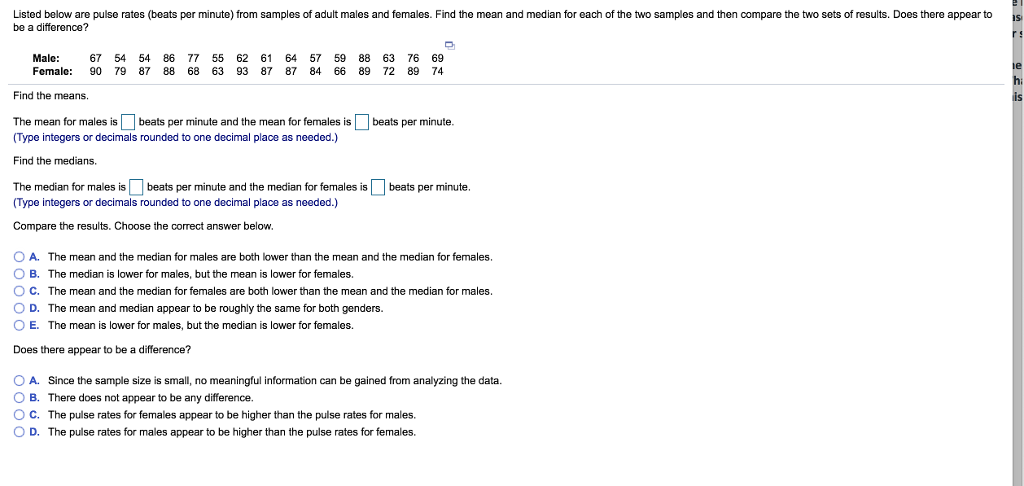Listed below are pulse rates (beats per minute) from samples of adult males and females. Find the mean and median for each of the two samples and then compare the two sets of results. Does there appear to be a difference? Male: 67 54 54 86 77 55 62 61 64 57 59 88 63 76 69 Female: 90 79 87 88 68 63 93 87 87 84 66 89 72 89 74 Find the means. The mean for males...

• ### Listed below are pulse rates (beats per minute) from samples of adult males and females. Find...Listed below are pulse rates (beats per minute) from samples of adult males and females. Find the mean and median for each of the two samples and then compare the two sets of results. Does there appear to be a difference? Male: 67 54 54 86 77 55 62 61 64 57 59 88 63 76 69 Female: 90 79 87 88 68 63 93 87 87 84 66 89 72 89 74 Find the means. The mean for males...

• ### on ма m Question Help Refer to the accompanying data set and construct a 90% confidence...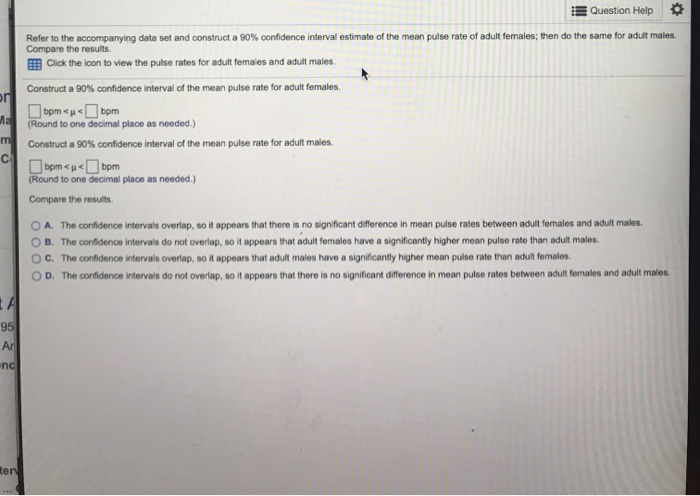on ма m Question Help Refer to the accompanying data set and construct a 90% confidence interval estimate of the mean pulse rate of adult females; then do the same for adult males. Compare the results. Click the icon to view the pulse rates for adult females and adult males Construct a 90% confidence interval of the mean pulse rate for adult females. bpmu<bpm (Round to one decimal place as needed.) Construct a 90% confidence interval of the mean pulse...

• ### Consider the below matrixA, which you can copy and paste directly into Matlab.

Problem #1: Consider the below matrix A, which you can copy and paste directly into Matlab. The matrix contains 3 columns. The first column consists of Test #1 marks, the second column is Test # 2 marks, and the third column is final exam marks for a large linear algebra course. Each row represents a particular student.A = [36 45 75 81 59 73 77 73 73 65 72 78 65 55 83 73 57 78 84 31 60 83...